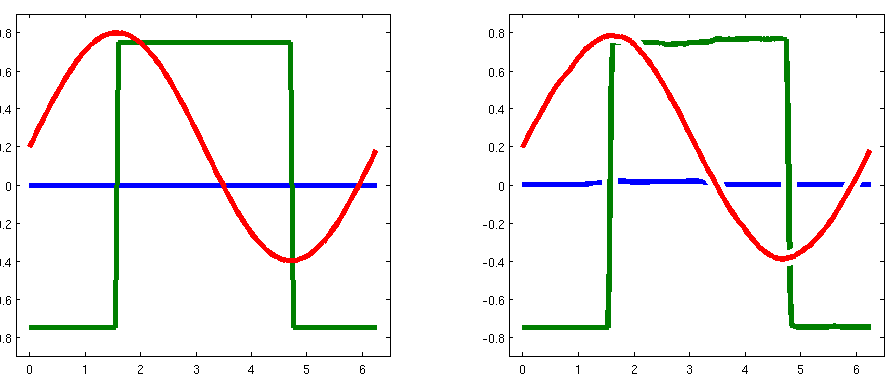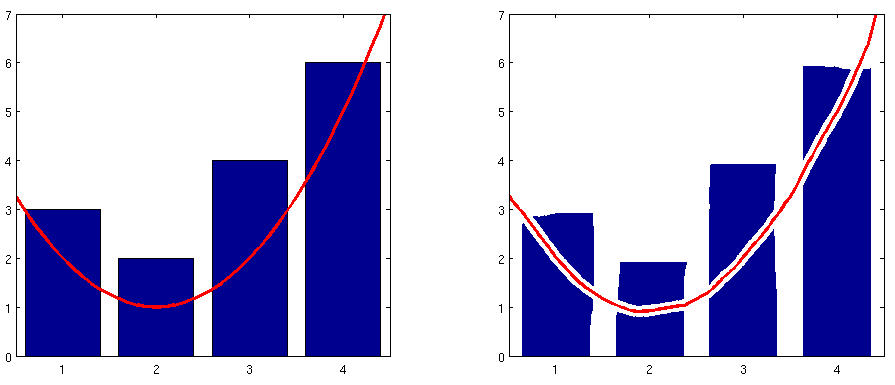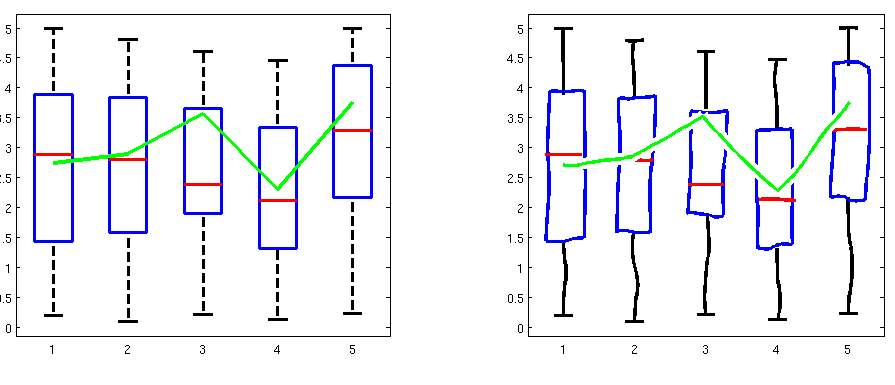Adding flair to boring Matlab Axes one plot at a time
You can not select more than 25 topics Topics must start with a letter or number, can include dashes ('-') and can be up to 35 characters long.Ben Lucht 0ea50238b9 Prefer xkcd-script font 4 years ago
11 years ago
11 years ago
11 years ago
11 years ago
11 years ago
11 years ago
4 years ago
11 years ago

# XKCDIFY

XKCDIFY is tool for converting most matlab plot to XKCD style plots!

XKCDIFY was designed to work in conjunction with the standard Matlab plotting utilities, making it compatible with most matlab code. Simply create plot as you would with any of the standard 2D plotting tools and when you satisfied you can then XKCDIFY them.

XKCDIFY works by iterating over the children of an axes and distorts the individual children based upon their TYPE. Currently only children of type LINE or PATCH get distorted. Additionally if the child type is HGGROUP then XKCDIFY iterates on the sub-children of the hggroup.

## Usage Examples Code

Here are a few examples of the types of plots that XKCDIFY can operate on. Each plot is drawn twice with the original on the left and the XKCD style plot on the right.

##### Simple Line Plots
``````figure('Position', [100 460 1120 420]);
a(1) = subplot(121); a(2) = subplot(122);

x = 0:.05:2*pi;
y1 = zeros(size(x));  % flat line
y2 =  mod(round(x / pi),2)*1.5 - .75; % Square wave
y3 = .2 + .6 * sin(x); % sine wave

plot(x,y1,x,y2,x,y3, 'linewidth', 4, 'Parent', a(1));
plot(x,y1,x,y2,x,y3, 'linewidth', 4, 'Parent', a(2));
set(a, 'XLim', [x(1) - .25, x(end)+.25], 'YLim', [-.9 .9]);

xkcdify(a(2));
``````##### Bar and Line Plots
``````%% - Example 2, XKCDify a bar plot with a line plot on top
clear; close all; clc;

figure('Position', [100 460 1120 420]);
a(1) = subplot(121); a(2) = subplot(122);

x = [0:.1:5];
y = 1 + (x-2).^2;

bar([ 3 2 4 6], 'Parent', a(1));
line(x,y,'Color', 'r', 'lineWidth', 3, 'Parent', a(1));
bar([ 3 2 4 6], 'Parent', a(2));
line(x,y,'Color', 'r', 'lineWidth', 3, 'Parent', a(2));
set(a, 'XLim', [.5 4.5], 'YLim', [0 7]);

xkcdify(a(2));
``````##### Box and Line Plots
``````clear; close all; clc;
n = 5;  data = rand(20,n) * 5;
x = 1:n; y =  mean(data) + rand(1,n);

figure('Position', [100 460 1120 420]);
a(1) = subplot(121); a(2) = subplot(122);

boxplot( data, 'Parent', a(1));

set( get(get(a(1), 'Children'),'Children'), 'LineWidth', 3); % Hack to grow the line width of the boxplot
line(x, y, 'color', 'g', 'linewidth', 3, 'Parent', a(1));

boxplot( data, 'Parent', a(2));

set( get(get(a(2), 'Children'),'Children'), 'LineWidth', 3); % Hack to grow the line width of the boxplot
line(x, y, 'color', 'g', 'linewidth', 3, 'Parent', a(2));

xkcdify(gca)
``````The source for these examples is found in xkcdify_examples.m